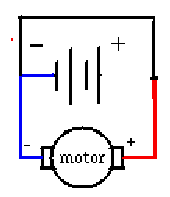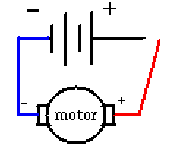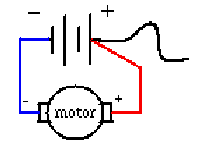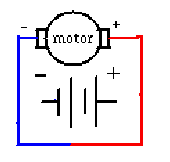Here's Four More To TryLook closely at this circuit.
1. Is there anything in this diagram that is incorrect ?
2. If you constructed this diagram using wire, lightbulb, a battery etc. will this circuit work?
1.
2.Look closely at this circuit.
1. Is there anything in this diagram that is incorrect ?
2. If you constructed this diagram using wire, lightbulb, a battery etc. will this circuit work?
3.Look closely at this circuit.
1. Is there anything in this diagram that is incorrect ?
2. If you constructed this diagram using wire, lightbulb, a battery etc. will this circuit work?
4.Look closely at this circuit.
1. Is there anything in this diagram that is incorrect ?
2. If you constructed this diagram using wire, lightbulb, a battery etc. will this circuit work?
BACK